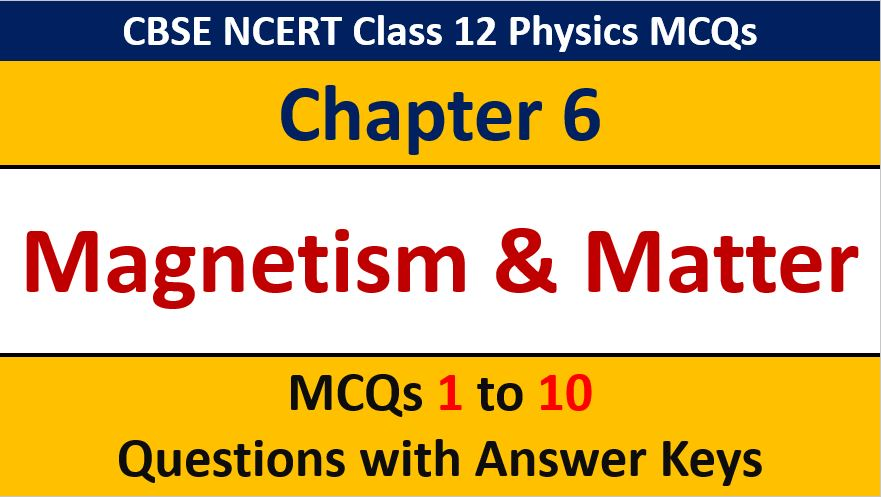## MCQ Questions for Class 12 Physics Chapter 6 Electromagnetic Induction with Answer Keys

If you are preparing for CBSE Class 12 Board Exam Term 1 which will be an objective type questions paper, then you need to practice multiple choice questions of Class 12 Physics Chapterwise. In this article of AKVTutorials, you will get MCQ Questions for Class 12 Physics Chapter 6 Electromagnetic Induction with Answer Keys.

MCQ Questions for Class 12 Physics Chapter 6 Question No 1

Lenz’s law is in accordance with the law of conservation of

Option A : Electric current

Option B : Energy

Option C : Electro motive force

Option D : Electric charge

Option B : Energy

MCQ Questions for Class 12 Physics Chapter 6 Question No 2:

When a metallic sphere is moved in a magnetic field, it gets heated due to

Option A : Air resistance

Option B : Induced currents called eddy currents

Option C : Current in the coil of magnet producing the field

Option D : Friction

Option B : Induced currents called eddy currents

MCQ Questions for Class 12 Physics Chapter 6 Question No 3:

Four solenoids having same area of cross section have the lengths and number of turns as in the choices given below. Which of them has the least coefficient of self induction? The one that is

Option A : Longer with more number of turns

Option B : Shorter with less number of turns

Option C : Shorter with more number of turns

Option D : Longer with less number of turns

Option D : Longer with less number of turns

MCQ Questions for Class 12 Physics Chapter 6 Question No 4:

Whenever the flux linked with a coil changes, then

Option A : An emf is always induced

Option B : An emf and a current are always induced

Option C : An emf is induced but a current is never induced

Option D : An emf is always induced and a current is induced, when the coil is a closed one

Option D : An emf is always induced and a current is induced, when the coil is a closed one

MCQ Questions for Class 12 Physics Chapter 6 Question No 5:

The laws of electromagnetic induction have been used in the construction of a

Option A : Galvanometer

Option B : Voltmeter

Option C : Electric motor

Option D : Electric generator

Option D : Electric generator

MCQ Questions for Class 12 Physics Chapter 6 Question No 6:

A metallic ring is attached to the wall of the room. When the north pole of magnet is brought near the ring, the induced current in the ring is

Option A : Zero

Option B : In clock-wise direction

Option C : In anti-clockwise direction

Option D : Infinite

Option C : In anti-clockwise direction

MCQ Questions for Class 12 Physics Chapter 6 Question No 7:

Consider the situation shown in the figure. If the current I in the long straight conducting wire XY is increased emf’s in loops A and B will be

Option A : Clockwise in A, anti-clockwise in B

Option B : Anticlockwise in A, clockwise in B

Option C : Clockwise in both A and B

Option D : Anticlockwise in both A and B

Option A : Clockwise in A, anti-clockwise in B

MCQ Questions for Class 12 Physics Chapter 6 Question No 8:

The direction of the induced emf is determined by

Option A : Fleming’s left hand rule

Option B : Lenz’s law

Option C : Maxwell’s right hand screw rule

Option D : Ampere’s rule of swimming

Option B : Lenz’s law

MCQ Questions for Class 12 Physics Chapter 6 Question No 9:

The coefficient of self inductance and the coefficient of mutual inductance have

Option A : Same units but different dimensions

Option B : Different units but same dimensions

Option C : Different units and different dimensions

Option D : Same units and same dimensions

Option D : Same units and same dimensions

MCQ Questions for Class 12 Physics Chapter 6 Question No 10:

A closed coil with a resistance R is placed in a magnetic field. The flux linked with the coil id f, if the magnetic field is suddenly reversed in direction, the charge that flows through the coil will be

Option A : f / 2R

Option B : f/ R

Option C : 2f / R

Option D : Zero

Option C : 2f / R

CBSE Class 12 Physics Chapterwise MCQ
1 : Electric Charge & Fields MCQs
| 1 to 10 | 11 to 20
2 : Electrostatics Potential and Capacitance MCQs
| 1 to 10 | 11 to 20
3 : Current Electricity MCQs
| 1 to 10 | 11 to 20
4 : Moving charges and Magnetism MCQs
| 1 to 10 | 11 to 20
5 : Magnetism and Matter MCQs
| 1 to 10 | 11 to 20
6 : Electromagnetic Induction MCQs
| 1 to 10 | 11 to 20
7 : Alternating Current MCQs
| 1 to 10 | 11 to 20
8 : Electromagnetic Waves MCQs
| 1 to 10 | 11 to 20
9 : Ray Optics and Optical Instruments MCQs
| 1 to 10 | 11 to 20
10 : Wave Optics MCQs
| 1 to 10 | 11 to 20
11 : Dual Nature of Radiation MCQs
| 1 to 10 | 11 to 20
12 : Atoms MCQs
| 1 to 10 | 11 to 20
13 : Nuclei MCQs
| 1 to 10 | 11 to 20
14 : Semi Conductor Electronics MCQs
| 1 to 10 | 11 to 20
15 : Communication Systems MCQs
| 1 to 10 | 11 to 20